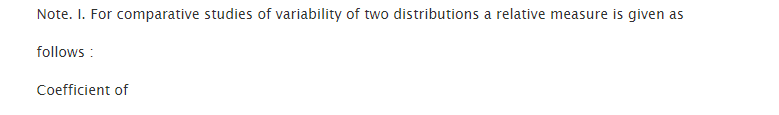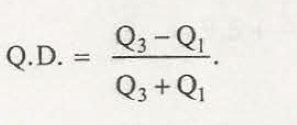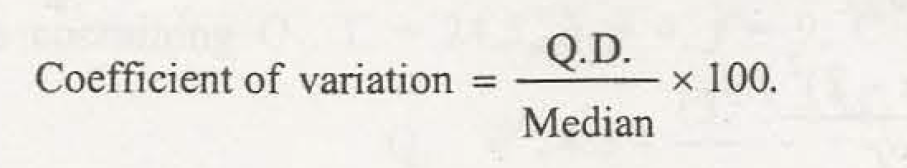It is actually based on lower quartile Q1 and upper quartile Q3. When there is a difference occurs between Q3 -Q1 then it is said to be inter quartile range. While difference between Q3 – Q1 divided by 2 is called semi inter-quartile range or even referred as quartile deviation. Therefore, it is defined as:

Q.D= Q3 -Q1/2

Quartile deviation is considered to be a better option that helps to measure absolute dispersion compared to range. But, this also ignores the observation on tails. Taking the difference of samples from population can enable you to calculate quartile deviations and the values may likely to be different. This is known as sampling fluctuation.

It is thought to be not so popular measure of dispersion. The quartile deviation can be calculated from sample data and this finally does not allow drawing any kind of conclusion related to quartile deviation in population.

Coefficient of quartile deviation

Relative measure of dispersion is actually based on quartile deviation which is finally termed as coefficient of quartile deviation. Therefore, this is described as:

Coefficient of quartile deviation = Q3 -Q1/ Q3 +Q1

Coefficient of quartile deviation
I. For relative educations of variability of dual distributions a comparative measure is given asII. If there is two much inconsistency between two supplies then the next measure is calculated :III. In the case of unequal class-intervals Q.D. is sometimes preferred to S.D.### Customer Reviews

My Homework Help
Rated 5.0 out of 5 based on 510 customer reviews at